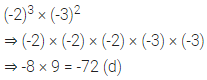# ML Aggarwal Class 6 Solutions for ICSE Maths Chapter 9 Algebra Objective Type Questions

## ML Aggarwal Class 6 Solutions for ICSE Maths Chapter 9 Algebra Objective Type Questions

Mental Maths
Question 1.
Fill in the blanks:
(i) In algebra, we use …………… to represent variables (generalized numbers).
(ii) A symbol or letter which can be given various numerical values is called a ……………
(iii) If Jaggu’s present age is x years, then his age 7 years from now is ……………
(iv) If one pen costs ₹X x, then the cost of 9 pens is ……………
(v) An equation is a statement that the two expressions are ……………
(vi) Trial an error is one of the methods to obtain …………… of an equation.
(vii) 7 less than thrice a number y is ……………
(viii) If 3x + 4 = 19, then the value of x is ……………
(ix) The number of pencils bought for ₹ x at the rate of ₹2 per pencil is ……………
(x) In the expression (-7)5, base = …………… and exponent = ……………
(xi) If base = 6 and exponent = 5, then the exponential form = …………… .
Solution: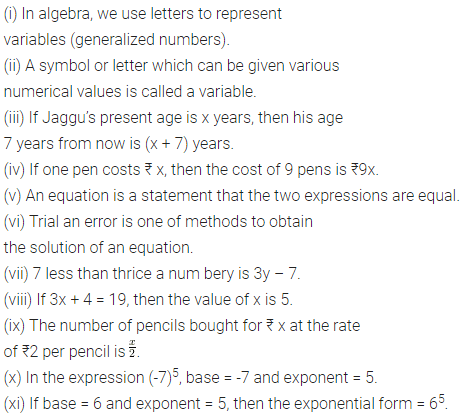Question 2.
State whether the following statements are true (T) or false (F):
(i) If x is variable then 5x is also variable.
(ii) If y is variable then y – 5 is also variable.
(iii) The number of angles in a triangle is a variable.
(iv) The value of an algebraic expression change with the change in the value of the variable.
(v) If the length of a rectangle is twice its breadth, then its area is a constant.
(vi) An equation is satisfied only for a definite value of the variable.
(vii) If x toffees are distributed equally among 5 children, then each child gets 5x toffees.
(viii) t minutes are equal to 60 t seconds.
(ix) If x is a negative integer, then -x is a positive integer.
(x) x = 5 is a solution of the equation 3x + 2 = 13.
(xi) 2y- 7 > 13 is an equation.
(xii) ‘One third of a number x added to itself gives 8’ can be expressed as $$\frac{x}{3}$$ + 8 = x.
(xiii)The difference between the ages of two sisters Lata and Asha is a variable.
Solution: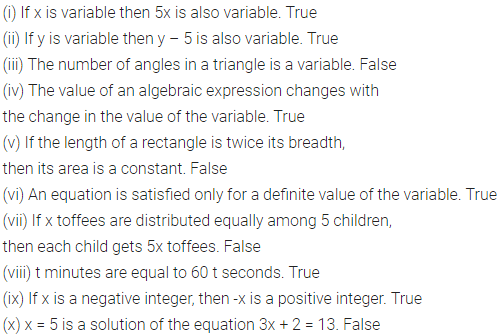Multiple Choice Questions
Choose the correct answer from the given four options (3 to 19):
Question 3.
I think of a number x, add 5 to it. The result is then multiplied by 2 and the final result is 24. The correct algebraic statement is
(a) x + 5 × 2 = 24
(b) (x + 5) × 2 = 24
(c) 2 × x + 5 = 24
(d) x + 5 = 2 × 24
Solution:Question 4.
Which of the following is an equation?
(a) x + 5
(b) 7x
(c) 2y + 3 = 11
(d) 2p < 1
Solution: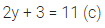Question 5.
If each matchbox contains 48 matchsticks, then the number of matchsticks required to fill n such boxes is
(i) 48 + n
(b) 48 – n
(c) 48 ÷ n
(d) 48n
Solution:Question 6.
If the perimeter of a regular hexagon is x metres, then the length of each of its sides is
(a) (x + 6) metres
(b) (x – 6) metres
(c) (x ÷ 6) metres
(d) (6 ÷ x) metres
Solution: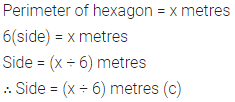Question 7.
x = 3 is the solution of the equation
(a) x + 7 = 4
(b) x + 10 = 7
(c) x + 7 = 10
(d) x + 3 = 7
Solution: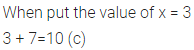Question 8.
The solution of the equation 3x – 2 = 10 is
(a) x = 1
(b) x = 2
(c) x = 3
(d) x = 4
Solution: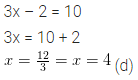Question 9.
The operation not involved in forming the expression 5x + $$\frac{5}{x}$$ from the variable x and number 5 is
(b) subtraction
(c) multiplication
(d) division
Solution: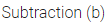Question 10.
The quotient of x by 3 added to 7 is written as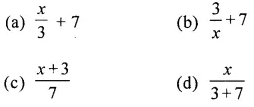Solution: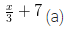Question 11.
If there are x chairs in a row, then the number of persons that can be seated in 8 rows are
(a) 64
(b) x + 8
(c) 8x
(d) none of these
Solution: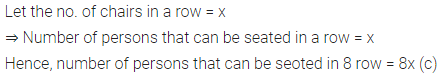Question 12.
If Arshad earns ₹ x per day and spends ₹ y per day, then his saving for the month of March is
(a) ₹(31x – y)
(b) ₹31(x – y)
(c) ₹31 (x + y)
(d) ₹31 (y – x)
Solution: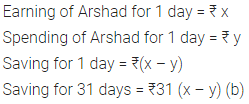Question 13.
If the length of a rectangle is 3 times its breadth and the breadth is x units, then its perimeter is
(a) 4x units
(b) 6x units
(c) 8x units
(d) 10x units
Solution: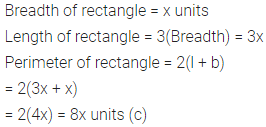Question 14.
Rashmi has a sum of ₹ x. She spend ₹800 on grocery, ₹600 on cloths and ₹500 on education and received as ₹200 as a gift. How much money (in ₹) is left with her?
(a) x – 1700
(b) x – 1900
(c) x + 200
(d) x – 2100
Solution:Question 15.
For any two integers a and b, which of the following suggests that the operation of addition is commutative?
(a) a × b = b × a
(b) a + b = b + a
(c) a – b = b – a
(d) a + b > a
Solution:Question 16.
In $$\left(\frac{3}{4}\right)^{5}$$, the base is
(a) 3
(b) 4
(c) 5
(d) $$\frac{3}{4}$$
Solution:Question 17.
a × a × b × b × b can be written as
(a) a2b3
(b) a3b2
(c) a3b3
(d) a5b5
Solution: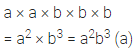Question 18.
(-5)2 × (-1)3 is equal to
(a) 25
(b) -25
(c) 10
(d) -10
Solution: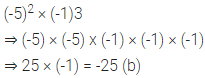Question 19.
(-2)3 × (-3)2 is equal to
(a) 65
(b) (-6)5
(c) 72
(d) -72
Solution: